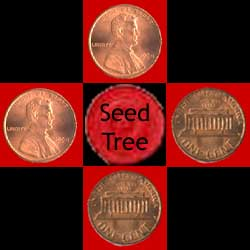HandsOn 28 - Growing a Forest from a Single Tree

An old, mighty oak tree stands alone in a field. Much of the ground is covered with randomly-scattered rocks. Autumn arrives, and the tree drops acorns on the ground nearby.

One acorn lands on a rock and dies. Another acorn lands on dirt, takes root, and a new tree grows.

The next autumn, every tree (both the original tree and all its "children'') drops acorns on the ground next to it. Again, an acorn that lands on a rock dies. An acorn that lands on an existing tree dies also. But an acorn that lands on dirt takes root and grows.

As the years go by, generation after generation of oaks spread away from the original mighty oak. If the area is rocky, with only small patches of exposed dirt, the trees probably won't spread very far. That's because the spreading cluster of oaks has to stop when it is surrounded on all sides by rocks. But if the area is not rocky, has lots of exposed dirt, the cluster of trees spreads and spreads. It does not stop until it reaches the edge of the field.

We start by growing a "forest'' by hand. Then we will go on to grow the forest using a computer simulation.

For this exercise, you might want to work with a partner. Each pair will need:

• a checkerboard,

• a set of red and black checkers, and

• a handful of pennies, 20 or so.

 Q7.9: Predict! What do you expect will happen? What will your pattern of trees look like when it is finished? Will your pattern of trees look exactly like everyone else's pattern? As your forest grows, do you think it will reach the edge? What might keep it from reaching the edge?

Now carry out the following steps:

1. Place a red checker on a square near the center of the checkerboard. This stands for the old, mighty oak whose acorns start the forest growth.

Now imagine that this tree drops acorns on the neighboring squares, up, down, right, and left. For each acorn, we must ask: does it grow into a new tree? Or is that square occupied by a big rock where no trees can grow?

Let's assume that for each neighboring square there is a 50% chance that a tree grows, and a 50% chance that the square contains a big rock. That is, the "tree probability'' is 50%, and the "rock probability'' is 50%.

2. Shake up four pennies between your cupped hands and, without looking at them, put one penny in each of the four neighboring squares right, left, up, and down (see Figure ).Figure 7.1: Checkerboard with 4 pennies and 1 checker.

3. Look at the four pennies. A head means a new tree; a tail means a rock. Replace each head with a red checker (tree), each tail with a black checker (rock).

4. Shake some pennies up again and place one in every empty square next to a red checker (tree) using the squares up, down, right, and left of the tree.

5. Again replace each head with a red checker (tree), and each tail with a black checker (rock).

6. Repeat steps 4 and 5 until the forest reaches the edge or cannot grow any further (all trees are surrounded by rocks).

On the board in front of the class, draw a table with two columns. Label the first column "Reach edge'' and the second column "Not reach edge.'' As each pair of students completes growing the forest, they report on whether or not their forest reached the edge. Enter their result in the table. Then each pair starts growing a new forest from the beginning. When each group has completed growing three forests, stop the activity and look at the table on the board. Have a discussion about the following questions:

 Q7.10: Why don't you get the same pattern every time?

 Q7.11: Why do some of the patterns reach the edge and others do not? Why don't they all behave the same?

 Q7.12: If we grew 1000 forests, what fraction of these forests would reach the edge? Do you have a definite percentage in mind?

 Q7.13: Would you expect the patterns to be different if we used a single die instead of a coin, and placed a tree on the site for numbers 1, 2, or 3 and a rock for numbers 4, 5, or 6? Would a greater or smaller fraction of the forests reach the edge than for the original game? Explain the reasoning behind your answer.

 Q7.14: Suppose we throw a single die and place a tree for number 1 but a rock for numbers 2, 3, 4, 5, or 6? In this case would a greater or smaller fraction of patterns reach the edge?

 Q7.15: Suppose we throw a die and place a tree on the site for numbers 1, 2, 3, 4, or 5 and a rock for number 6 only. How will this change the pattern of the forest? Will it increase or decrease the chance of the forest reaching the edge?

 Q7.16: When the tree probability drops from 0.5 to 0.1, is the forest more likely or less likely to reach the edge? What about when the tree probability rises from 0.5 to 0.9. Is growth to the edge more likely or less likely in this case? Suppose we grow many forests, each with a different tree probability, starting at 0.1 and increasing to 0.9. Will the fraction reaching the edge increase or decrease as tree probability increases? Will this increase or decrease take place gradually as probabilities get bigger or suddenly at a particular probability?

Next: SimuLab 18 - Percolating Forests